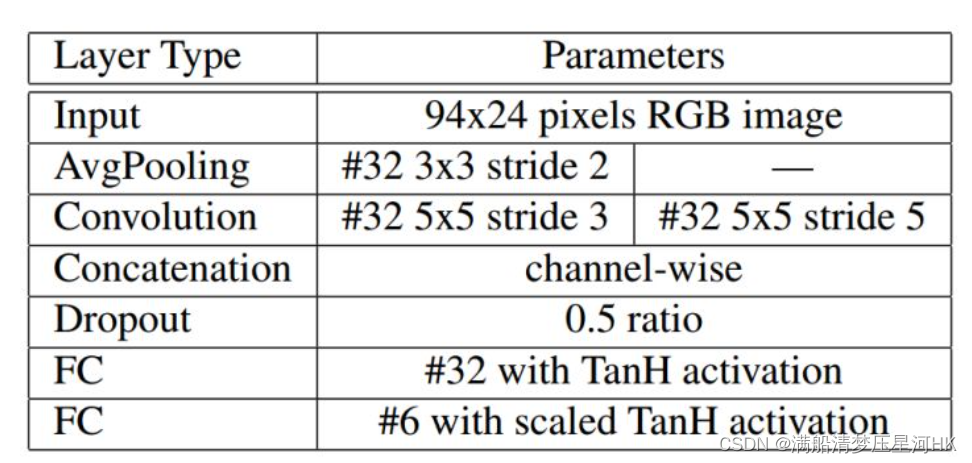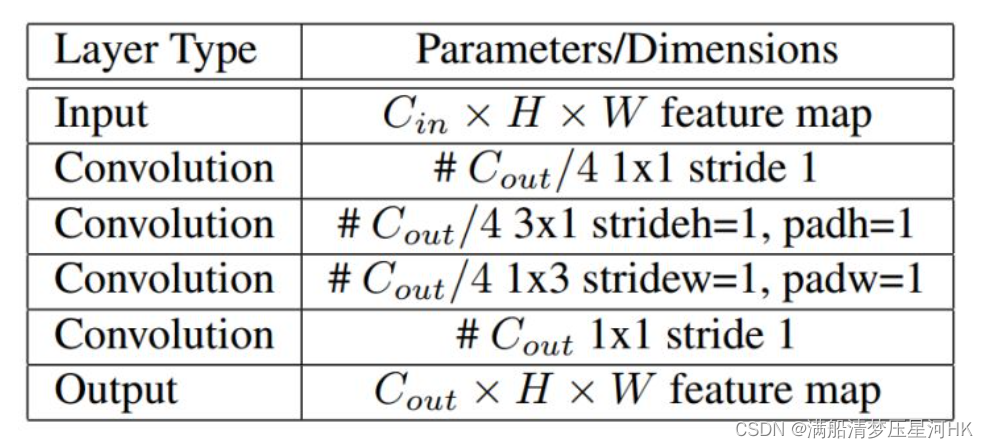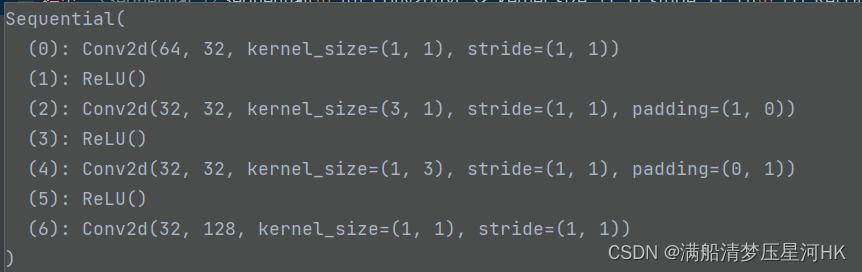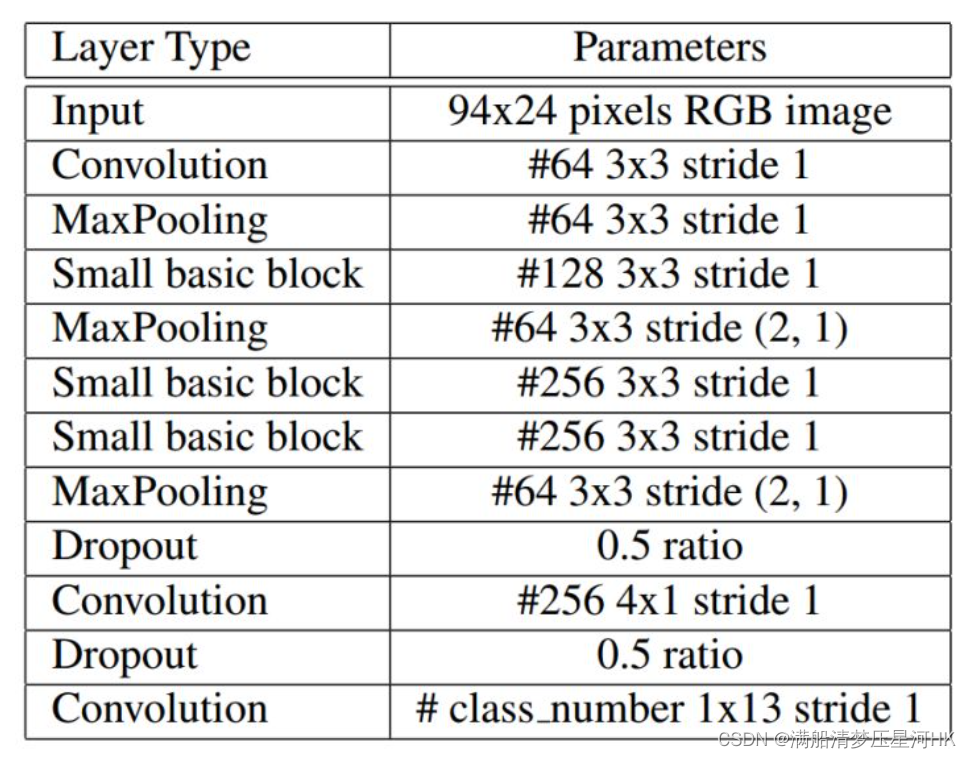0

# 【项目三、车牌检测+识别项目】三、LPRNet车牌识别网络原理和核心源码解读

## 前言

1. 【项目一、xxx病虫害检测项目】1、SSD原理和源码分析
2. 【项目一、xxx病虫害检测项目】2、网络结构尝试改进：Resnet50、SE、CBAM、Feature Fusion
3. 【项目一、xxx病虫害检测项目】3、损失函数尝试：Focal loss

【项目二、蜂巢检测项目】一、串讲各类经典的卷积网络：InceptionV1-V4、ResNetV1-V2、MobileNetV1-V3、ShuffleNetV1-V2、ResNeXt、Xception。
【项目二、蜂巢检测项目】二、模型改进：YOLOv5s-ShuffleNetV2。

1. 【项目三、车牌检测+识别项目】一、CCPD车牌数据集转为YOLOv5格式和LPRNet格式
2. 【项目三、车牌检测+识别项目】二、使用YOLOV5进行车牌检测
3. 【项目三、车牌检测+识别项目】三、LPRNet车牌识别网络原理和核心源码解读
4. 【项目三、车牌检测+识别项目】四、使用LPRNet进行车牌识别

## 一、LPRNet网络介绍

LPRNet是一个非常经典的车牌识别算法，论文是Intel于2018年发表的： LPRNet: License Plate Recognition via Deep Neural Networks，整个网络结构设计高度轻量化，只用1.5M，瞄准的就是在嵌入式设备中使用，但是识别率却毫不逊色，Intel已经在自己的嵌入式设备中应用了。

1. 不需要对字符进行预分割，是一个端到端的轻量化字符识别模型，速度快，精度还不错；这里主要是因为仿照squeezenet和inception的思想设计了一个轻量化的卷积模块。
3. 损失使用的CTC Loss、推理应用了贪心算法，搜索取每个位置上类概率的最大值。

## 2.1、STN定位网络## 2.2、BackboneLPRNet的backbone模块是自行设计的一个轻量化的backbone，其中自己设计的一个Small basic block其实就是一个瓶颈型的结构（有点像去掉shortcut分枝的bottleneck），下面来好好说说。

Small basic block结构如下（思路：squeezenet/bottleneck+inception）：``````classsmall_basic_block(nn.Module):def__init__(self, ch_in, ch_out):super(small_basic_block, self).__init__()
self.block = nn.Sequential(
nn.Conv2d(ch_in, ch_out //4, kernel_size=1),
nn.ReLU(),
nn.Conv2d(ch_out //4, ch_out //4, kernel_size=(3,1), padding=(1,0)),
nn.ReLU(),
nn.Conv2d(ch_out //4, ch_out //4, kernel_size=(1,3), padding=(0,1)),
nn.ReLU(),
nn.Conv2d(ch_out //4, ch_out, kernel_size=1),)defforward(self, x):return self.block(x)
``````

backbone的整体架构：``````# __init__
self.backbone = nn.Sequential(
nn.Conv2d(in_channels=3, out_channels=64, kernel_size=3, stride=1),# 0  [bs,3,24,94] -> [bs,64,22,92]
nn.BatchNorm2d(num_features=64),# 1  -> [bs,64,22,92]
nn.ReLU(),# 2  -> [bs,64,22,92]
nn.MaxPool3d(kernel_size=(1,3,3), stride=(1,1,1)),# 3  -> [bs,64,20,90]
small_basic_block(ch_in=64, ch_out=128),# 4  -> [bs,128,20,90]
nn.BatchNorm2d(num_features=128),# 5  -> [bs,128,20,90]
nn.ReLU(),# 6  -> [bs,128,20,90]
nn.MaxPool3d(kernel_size=(1,3,3), stride=(2,1,2)),# 7  -> [bs,64,18,44]
small_basic_block(ch_in=64, ch_out=256),# 8  -> [bs,256,18,44]
nn.BatchNorm2d(num_features=256),# 9  -> [bs,256,18,44]
nn.ReLU(),# 10 -> [bs,256,18,44]
small_basic_block(ch_in=256, ch_out=256),# 11 -> [bs,256,18,44]
nn.BatchNorm2d(num_features=256),# 12 -> [bs,256,18,44]
nn.ReLU(),# 13 -> [bs,256,18,44]
nn.MaxPool3d(kernel_size=(1,3,3), stride=(4,1,2)),# 14 -> [bs,64,16,21]
nn.Dropout(dropout_rate),# 0.5 dropout rate                          # 15 -> [bs,64,16,21]
nn.Conv2d(in_channels=64, out_channels=256, kernel_size=(1,4), stride=1),# 16 -> [bs,256,16,18]
nn.BatchNorm2d(num_features=256),# 17 -> [bs,256,16,18]
nn.ReLU(),# 18 -> [bs,256,16,18]
nn.Dropout(dropout_rate),# 0.5 dropout rate                                  19 -> [bs,256,16,18]
nn.Conv2d(in_channels=256, out_channels=class_num, kernel_size=(13,1), stride=1),# class_num=68  20  -> [bs,68,4,18]
nn.BatchNorm2d(num_features=class_num),# 21 -> [bs,68,4,18]
nn.ReLU(),# 22 -> [bs,68,4,18])# __forward__for i, layer inenumerate(self.backbone.children()):
x = layer(x)
``````

## 2.3、全局上下文嵌入

``````        global_context =list()# keep_features: [bs,64,22,92]  [bs,128,20,90] [bs,256,18,44] [bs,68,4,18]for i, f inenumerate(keep_features):if i in[0,1]:# [bs,64,22,92] -> [bs,64,4,18]# [bs,128,20,90] -> [bs,128,4,18]
f = nn.AvgPool2d(kernel_size=5, stride=5)(f)if i in:# [bs,256,18,44] -> [bs,256,4,18]
f = nn.AvgPool2d(kernel_size=(4,10), stride=(4,2))(f)
f_pow = torch.pow(f,2)
f_mean = torch.mean(f_pow)
f = torch.div(f, f_mean)
global_context.append(f)

x = torch.cat(global_context,1)
``````

``````# __init__
self.container = nn.Sequential(
nn.Conv2d(in_channels=448+self.class_num, out_channels=self.class_num, kernel_size=(1,1), stride=(1,1)),# nn.BatchNorm2d(num_features=self.class_num),# nn.ReLU(),# nn.Conv2d(in_channels=self.class_num, out_channels=self.lpr_max_len+1, kernel_size=3, stride=2),# nn.ReLU(),)# __forward__
x = self.container(x)# -> [bs, 68, 4, 18]   head头
logits = torch.mean(x, dim=2)# -> [bs, 68, 18]  # 68 字符类别数   18字符序列长度return logits
``````

## 三、CTC Loss

``````ctc_loss = nn.CTCLoss(blank=len(CHARS)-1, reduction='mean')# reduction: 'none' | 'mean' | 'sum'...# 网络输出 [bs,68,18]# log_probs: 预测结果 [18, bs, 68]  其中18为序列长度  68为字典数# labels:   # input_lengths:  tuple   example: 000=18  001=18...   每个序列长度 # target_lengths: tuple   example: 000=7   001=8 ...   每个gt长度
loss = ctc_loss(log_probs, labels, input_lengths=input_lengths, target_lengths=target_lengths)
``````

## 四、后处理-解码

``````defGreedy_Decode_Eval(Net, datasets, args):# TestNet = Net.eval()
epoch_size =len(datasets)// args.test_batch_size
batch_iterator =iter(DataLoader(datasets, args.test_batch_size, shuffle=True, num_workers=args.num_workers, collate_fn=collate_fn))

Tp =0
Tn_1 =0
Tn_2 =0
t1 = time.time()for i inrange(epoch_size):# load train data
images, labels, lengths =next(batch_iterator)
start =0
targets =[]for length in lengths:
label = labels[start:start+length]
targets.append(label)
start += length
targets = np.array([el.numpy()for el in targets])
imgs = images.numpy().copy()if args.cuda:
images = Variable(images.cuda())else:
images = Variable(images)# forward# images: [bs, 3, 24, 94]# prebs:  [bs, 68, 18]
prebs = Net(images)# greedy decode
prebs = prebs.cpu().detach().numpy()
preb_labels =list()for i inrange(prebs.shape):
preb = prebs[i,:,:]# 对每张图片 [68, 18]
preb_label =list()for j inrange(preb.shape):# 18  返回序列中每个位置最大的概率对应的字符idx  其中'-'是67
preb_label.append(np.argmax(preb[:, j], axis=0))
no_repeat_blank_label =list()
pre_c = preb_labelif pre_c !=len(CHARS)-1:# 记录重复字符
no_repeat_blank_label.append(pre_c)for c in preb_label:# 去除重复字符和空白字符'-'if(pre_c == c)or(c ==len(CHARS)-1):if c ==len(CHARS)-1:
pre_c = c
continue
no_repeat_blank_label.append(c)
pre_c = c
preb_labels.append(no_repeat_blank_label)# 得到最终的无重复字符和无空白字符的序列for i, label inenumerate(preb_labels):# 统计准确率# show image and its predict labelif args.show:
show(imgs[i], label, targets[i])iflen(label)!=len(targets[i]):
Tn_1 +=1# 错误+1continueif(np.asarray(targets[i])== np.asarray(label)).all():
Tp +=1# 完全正确+1else:
Tn_2 +=1
Acc = Tp *1.0/(Tp + Tn_1 + Tn_2)print("[Info] Test Accuracy: {} [{}:{}:{}:{}]".format(Acc, Tp, Tn_1, Tn_2,(Tp+Tn_1+Tn_2)))
t2 = time.time()print("[Info] Test Speed: {}s 1/{}]".format((t2 - t1)/len(datasets),len(datasets)))
``````

## 五、其他注意的点

1. 网络输入只能是 bsx3x24x94 ，对应网络输出是 bsx68x18，其中68是字典中字符总个数，18个预测序列长度；

## 六、总结

2. 网络之所以非常前量化，主要是因为backbone设计了一种超轻量的模块（Small basic block），灵感来源于SqueezeNet和Inception，先用1x1卷积进行降维，再接一个3x1和1x3卷积代替原先的3x3卷积进行特征提取，在效果差不多的情况下，不但可以降低模型参数量还能增加非线性表达。最后再接一个1x1卷积进行升维。
3. 损失使用的CTC Loss、推理应用了贪心算法，搜索取每个位置上类概率的最大值。

## Reference

CSDN: linux-mobaxterm-yolov5训练数据集ccpd–无数踩雷后

### “【项目三、车牌检测+识别项目】三、LPRNet车牌识别网络原理和核心源码解读”的评论:

##### 关于作者##### overfit同步小助手